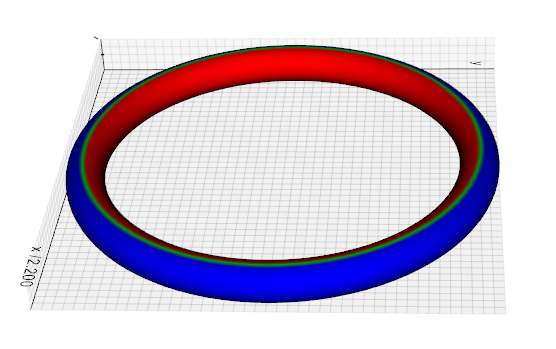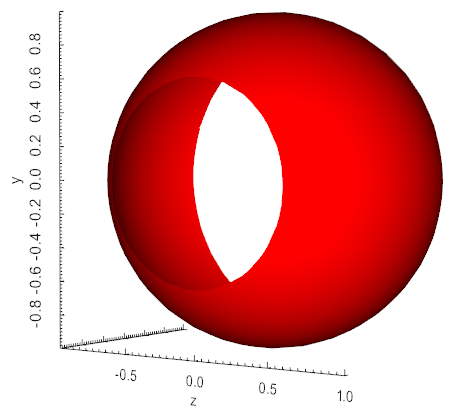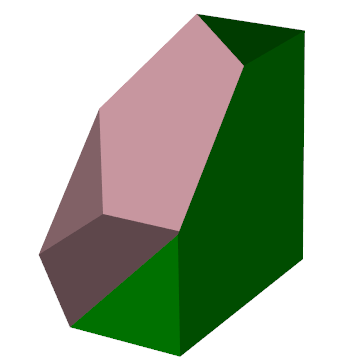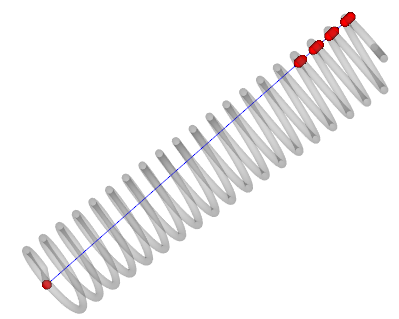# mesh¶

Submodule to manage polygonal meshes.

## Mesh¶

class vedo.mesh.Mesh(inputobj=None, c=None, alpha=1, computeNormals=False)[source]

Build an instance of object Mesh derived from PointCloud.

Input can be vtkPolyData, vtkActor, or a python list of [vertices, faces].

If input is any of vtkUnstructuredGrid, vtkStructuredGrid or vtkRectilinearGrid the geometry is extracted. In this case the original VTK data structures can be accessed with: mesh.inputdata().

Finally input can be a list of vertices and their connectivity (faces of the polygonal mesh). For point clouds - e.i. no faces - just substitute the faces list with None.

E.g.: Mesh( [ [[x1,y1,z1],[x2,y2,z2], …], [[0,1,2], [1,2,3], …] ] )

Parameters
• c – color in RGB format, hex, symbol or name

• alpha (float) – opacity value

• wire (bool) – show surface as wireframe

• bc – backface color of internal surface

• texture (str) – jpg file name or surface texture name

• computeNormals (bool) – compute point and cell normals at creation

Hint

A mesh can be built from vertices and their connectivity. See e.g.:

addCurvatureScalars(method=0, lut=None)[source]

Add scalars to Mesh that contains the curvature calculated in three different ways.

Parameters
• method (int) – 0-gaussian, 1-mean, 2-max, 3-min curvature.

• lut – optional vtkLookUpTable up table.

Example
from vedo import TorusaddElevationScalars(lowPoint=(), highPoint=(), vrange=(), lut=None)[source]

Add to Mesh a scalar array that contains distance along a specified direction.

Parameters
• low (list) – one end of the line (small scalar values). Default (0,0,0).

• high (list) – other end of the line (large scalar values). Default (0,0,1).

• vrange (list) – set the range of the scalar. Default is (0, 1).

• lut – optional vtkLookUpTable up table (see makeLUT method).

Example
from vedo import Sphere

|elevation|

addShadow(x=None, y=None, z=None, c=0.5, 0.5, 0.5, alpha=1, culling=1)[source]

Generate a shadow out of an Mesh on one of the three Cartesian planes. The output is a new Mesh representing the shadow. This new mesh is accessible through mesh.shadow. By default the shadow mesh is placed on the bottom/back wall of the bounding box.

Parameters
• x,y,z (float) – identify the plane to cast the shadow to [‘x’, ‘y’ or ‘z’]. The shadow will lay on the orthogonal plane to the specified axis at the specified value of either x, y or z.

• culling (int) – choose between front  or backface [-1] culling or None.

area(value=None)[source]

Get/set the surface area of mesh.

backColor(bc=None)[source]

Set/get mesh’s backface color.

backFaceCulling(value=True)[source]

Set culling of polygons based on orientation of normal with respect to camera.

bc(backColor=False)[source]

Shortcut for mesh.backColor().

boolean(operation, mesh2)[source]

Volumetric union, intersection and subtraction of surfaces.

Parameters

operation (str) – allowed operations: 'plus', 'intersect', 'minus'.

boundaries(boundaryEdges=True, featureAngle=65, nonManifoldEdges=True, returnPointIds=False, returnCellIds=False)[source]

Return a Mesh that shows the boundary lines of an input mesh.

Parameters
• boundaryEdges (bool) – Turn on/off the extraction of boundary edges.

• featureAngle (float) – Specify the feature angle for extracting feature edges.

• nonManifoldEdges (bool) – Turn on/off the extraction of non-manifold edges.

• returnPointIds (bool) – return a numpy array of point indices

• returnCellIds (bool) – return a numpy array of cell indices

cap(returnCap=False)[source]

Generate a “cap” on a clipped mesh, or caps sharp edges.

clone()[source]

Clone a Mesh object to make an exact copy of it.

computeNormals(points=True, cells=True)[source]

Compute cell and vertex normals for the mesh.

Warning

Mesh gets modified, output can have a different nr. of vertices.

connectedCells(index, returnIds=False)[source]

Find all cellls connected to an input vertex specified by its index.

connectedVertices(index, returnIds=False)[source]

Find all vertices connected to an input vertex specified by its index.

Parameters

returnIds (bool) – return vertex IDs instead of vertex coordinates.

crop(top=None, bottom=None, right=None, left=None, front=None, back=None, bounds=None)[source]

Crop an Mesh object.

Parameters
• top (float) – fraction to crop from the top plane (positive z)

• bottom (float) – fraction to crop from the bottom plane (negative z)

• front (float) – fraction to crop from the front plane (positive y)

• back (float) – fraction to crop from the back plane (negative y)

• right (float) – fraction to crop from the right plane (positive x)

• left (float) – fraction to crop from the left plane (negative x)

• bounds (list) – direct list of bounds passed as [x0,x1, y0,y1, z0,z1]

Example

from vedo import Sphere
Sphere().crop(right=0.3, left=0.1).show()cutWithMesh(mesh, invert=False)[source]

Cut an Mesh mesh with another Mesh.

Parameters

invert (bool) – if True return cut off part of Mesh.

cutWithPlane(origin=0, 0, 0, normal=1, 0, 0, returnCut=False)[source]

Cut the mesh with the plane defined by a point and a normal.

Parameters
• origin – the cutting plane goes through this point

• normal – normal of the cutting plane

Example
from vedo import Cube

cube = Cube().cutWithPlane(normal=(1,1,1))
cube.bc('pink').show()cutWithPointLoop(points, invert=False)[source]

Cut an Mesh object with a set of points forming a closed loop.

decimate(fraction=0.5, N=None, method='quadric', boundaries=False)[source]

Downsample the number of vertices in a mesh to fraction.

Parameters
• fraction (float) – the desired target of reduction.

• N (int) – the desired number of final points (fraction is recalculated based on it).

• method (str) – can be either ‘quadric’ or ‘pro’. In the first case triagulation will look like more regular, irrespective of the mesh origianl curvature. In the second case triangles are more irregular but mesh is more precise on more curved regions.

• boundaries (bool) – (True), in pro mode decide whether to leave boundaries untouched or not.

Note

Setting fraction=0.1 leaves 10% of the original nr of vertices.

distanceToMesh(mesh, signed=False, negate=False)[source]

Computes the (signed) distance from one mesh to another.

extractLargestRegion()[source]

Extract the largest connected part of a mesh and discard all the smaller pieces.

extrude(zshift=1, rotation=0, dR=0, cap=True, res=1)[source]

Sweep a polygonal data creating a “skirt” from free edges and lines, and lines from vertices. The input dataset is swept around the z-axis to create new polygonal primitives. For example, sweeping a line results in a cylindrical shell, and sweeping a circle creates a torus.

You can control whether the sweep of a 2D object (i.e., polygon or triangle strip) is capped with the generating geometry. Also, you can control the angle of rotation, and whether translation along the z-axis is performed along with the rotation. (Translation is useful for creating “springs”). You also can adjust the radius of the generating geometry using the “dR” keyword.

The skirt is generated by locating certain topological features. Free edges (edges of polygons or triangle strips only used by one polygon or triangle strips) generate surfaces. This is true also of lines or polylines. Vertices generate lines.

This filter can be used to model axisymmetric objects like cylinders, bottles, and wine glasses; or translational/rotational symmetric objects like springs or corkscrews.

Warning

Some polygonal objects have no free edges (e.g., sphere). When swept, this will result in two separate surfaces if capping is on, or no surface if capping is off.

faces()[source]

Get cell polygonal connectivity ids as a python list. The output format is: [[id0 … idn], [id0 … idm], etc].

fillHoles(size=None)[source]

Identifies and fills holes in input mesh. Holes are identified by locating boundary edges, linking them together into loops, and then triangulating the resulting loops.

Parameters

size (float) – approximate limit to the size of the hole that can be filled.

Example: fillholes.py

flat()[source]

Set surface interpolation to Flat.followCamera(cam=None)[source]

Mesh object will follow camera movements and stay locked to it. Use mesh.followCamera(False) to disable it.

Parameters

cam (vtkCamera) – if None the text will auto-orient itself to the active camera. A vtkCamera object can also be passed.

frontFaceCulling(value=True)[source]

Set culling of polygons based on orientation of normal with respect to camera.

geodesic(start, end)[source]

Dijkstra algorithm to compute the geodesic line. Takes as input a polygonal mesh and performs a single source shortest path calculation.

Parameters
• start (int, list) – start vertex index or close point [x,y,z]

• end – end vertex index or close point [x,y,z]

implicitModeller(distance=0.05, res=50, 50, 50, bounds=(), maxdist=None, outer=True)[source]

Find the surface which sits at the specified distance from the input one.

insidePoints(pts, invert=False, tol=1e-05, returnIds=False)[source]

Return the point cloud that is inside mesh surface.

intersectWith(mesh2, tol=1e-06)[source]

Intersect this Mesh with the input surface to return a line.

intersectWithLine(p0, p1)[source]

Return the list of points intersecting the mesh along the segment defined by two points p0 and p1.

Example
from vedo import *
s = Spring(alpha=0.2)
pts = s.intersectWithLine([0,0,0], [1,0.1,0])
ln = Line([0,0,0], [1,0.1,0], c='blue')
ps = Points(pts, r=10, c='r')
show(s, ln, ps, bg='white')isInside(point, tol=0.0001)[source]

Return True if point is inside a polydata closed surface.

isobands(n=10, vmin=None, vmax=None)[source]

Return a new Mesh representing the isobands of the active scalars. This is a new mesh where the scalar is now associated to cell faces and used to colorize the mesh.

Parameters
• n (int) – number of isolines in the range

• vmin (float) – minimum of the range

• vmax (float) – maximum of the range

isolines(n=10, vmin=None, vmax=None)[source]

Return a new Mesh representing the isolines of the active scalars.

Parameters
• n (int) – number of isolines in the range

• vmin (float) – minimum of the range

• vmax (float) – maximum of the range

join(polys=True)[source]

Generate triangle strips and/or polylines from input polygons, triangle strips, and lines.

Input polygons are assembled into triangle strips only if they are triangles; other types of polygons are passed through to the output and not stripped. Use mesh.triangulate() to triangulate non-triangular polygons prior to running this filter if you need to strip all the data.

Also note that if triangle strips or polylines are present in the input they are passed through and not joined nor extended. If you wish to strip these use mesh.triangulate() to fragment the input into triangles and lines prior to applying strip().

Parameters

polys (bool) – polygonal segments will be joined if they are contiguous

Warning

If triangle strips or polylines exist in the input data they will be passed through to the output data. This filter will only construct triangle strips if triangle polygons are available; and will only construct polylines if lines are available.

lc(lineColor=None)[source]

Set/get color of mesh edges. Same as lineColor().

lineColor(lc=None)[source]

Set/get color of mesh edges. Same as lc().

lineWidth(lw=None)[source]

Set/get width of mesh edges. Same as lw().

lines(flat=False)[source]

Get lines connectivity ids as a numpy array. Default format is [[id0,id1], [id3,id4], …]

Parameters

flat (bool) – 1D numpy array as [2, 10,20, 3, 10,11,12, 2, 70,80, …]

lw(lineWidth=None)[source]

Set/get width of mesh edges. Same as lineWidth().

phong()[source]

Set surface interpolation to Phong.

quality(measure=6, cmap='RdYlBu')[source]

Calculate functions of quality of the elements of a triangular mesh. See class vtkMeshQuality for explanation.

Parameters

measure (int) –

type of estimator

• EDGE RATIO, 0

• ASPECT RATIO, 1

• ASPECT FROBENIUS, 3

• MED ASPECT_FROBENIUS, 4

• MAX ASPECT FROBENIUS, 5

• MIN_ANGLE, 6

• COLLAPSE RATIO, 7

• MAX ANGLE, 8

• CONDITION, 9

• SCALED JACOBIAN, 10

• SHEAR, 11

• RELATIVE SIZE SQUARED, 12

• SHAPE, 13

• SHAPE AND SIZE, 14

• DISTORTION, 15

• MAX EDGE RATIO, 16

• SKEW, 17

• TAPER, 18

• VOLUME, 19

• STRETCH, 20

• DIAGONAL, 21

• DIMENSION, 22

• ODDY, 23

• SHEAR AND SIZE, 24

• JACOBIAN, 25

• WARPAGE, 26

• ASPECT GAMMA, 27

• AREA, 28

• ASPECT BETA, 29

reverse(cells=True, normals=False)[source]

Reverse the order of polygonal cells and/or reverse the direction of point and cell normals. Two flags are used to control these operations:

• cells=True reverses the order of the indices in the cell connectivity list.

• normals=True reverses the normals by multiplying the normal vector by -1

(both point and cell normals, if present).

shrink(fraction=0.85)[source]

Shrink the triangle polydata in the representation of the input mesh.

Example

from vedo import *
s = Sphere(r=0.2).pos(0,0,-0.5)
show(pot, s)

silhouette(direction=None, borderEdges=True, featureAngle=False)[source]

Return a new line Mesh which corresponds to the outer silhouette of the input as seen along a specified direction, this can also be a vtkCamera object.

Parameters
• direction (list) – viewpoint direction vector. If None this is guessed by looking at the minimum of the sides of the bounding box.

• borderEdges (bool) – enable or disable generation of border edges

• featureAngle (float) – minimal angle for sharp edges detection. If set to False the functionality is disabled.

smoothLaplacian(niter=15, relaxfact=0.1, edgeAngle=15, featureAngle=60)[source]

Adjust mesh point positions using Laplacian smoothing.

Parameters
• niter (int) – number of iterations.

• relaxfact (float) – relaxation factor. Small relaxfact and large niter are more stable.

• edgeAngle (float) – edge angle to control smoothing along edges (either interior or boundary).

• featureAngle (float) – specifies the feature angle for sharp edge identification.

smoothWSinc(niter=15, passBand=0.1, edgeAngle=15, featureAngle=60)[source]

Adjust mesh point positions using the Windowed Sinc function interpolation kernel.

Parameters
• niter (int) – number of iterations.

• passBand (float) – set the passband value for the windowed sinc filter.

• edgeAngle (float) – edge angle to control smoothing along edges (either interior or boundary).

• featureAngle (float) – specifies the feature angle for sharp edge identification.

splitByConnectivity(maxdepth=1000)[source]

Split a mesh by connectivity and order the pieces by increasing area.

Parameters

maxdepth (int) – only consider this number of mesh parts.

stretch(q1, q2)[source]

Stretch mesh between points q1 and q2. Mesh is not affected.

Note

for Mesh objects like helices, Line, cylinders, cones etc., two attributes mesh.base, and mesh.top are already defined.

subdivide(N=1, method=0)[source]

Increase the number of vertices of a surface mesh.

Parameters
• N (int) – number of subdivisions.

• method (int) – Loop(0), Linear(1), Adaptive(2), Butterfly(3)

texture(tname, tcoords=None, interpolate=True, repeat=True, edgeClamp=False, scale=None, ushift=None, vshift=None)[source]

Assign a texture to mesh from image file or predefined texture tname. If tname is None texture is disabled. Input tname can also be an array of shape (n,m,3).

Parameters
• interpolate (bool) – turn on/off linear interpolation of the texture map when rendering.

• repeat (bool) – repeat of the texture when tcoords extend beyond the [0,1] range.

• edgeClamp (bool) – turn on/off the clamping of the texture map when the texture coords extend beyond the [0,1] range. Only used when repeat is False, and edge clamping is supported by the graphics card.

• scale (bool) – scale the texture image by this factor

• ushift (bool) – shift u-coordinates of texture by this amaount

• vshift (bool) – shift v-coordinates of texture by this amaount

threshold(scalars, above=None, below=None, useCells=False)[source]

Extracts cells where scalar value satisfies threshold criterion.

Parameters
• scalars (str,list) – name of the scalars array.

• above (float) – minimum value of the scalar

• below (float) – maximum value of the scalar

• useCells (bool) – if True, assume array scalars refers to cells.

triangulate(verts=True, lines=True)[source]

Converts mesh polygons into triangles.

If the input mesh is only made of 2D lines (no faces) the output will be a triangulation that fills the internal area. The contours may be concave, and may even contain holes, i.e. a contour may contain an internal contour winding in the opposite direction to indicate that it is a hole.

Parameters
• verts (bool) – if True, break input vertex cells into individual vertex cells (one point per cell). If False, the input vertex cells will be ignored.

• lines (bool) – if True, break input polylines into line segments. If False, input lines will be ignored and the output will have no lines.

volume(value=None)[source]

Get/set the volume occupied by mesh.

wireframe(value=True)[source]

Set mesh’s representation as wireframe or solid surface. Same as mesh.wireframe().

## merge¶

vedo.mesh.merge(*meshs)[source]

Build a new mesh formed by the fusion of the polygonal meshes of the input objects. Similar to Assembly, but in this case the input objects become a single mesh.